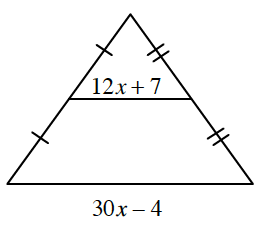### Home > INT1 > Chapter Ch11 > Lesson 11.2.4 > Problem11-93

11-93.

Write an equation and solve for $x$.

Although you do not have dimensions, you can write an equation by examining the relationship between the side lengths.

$2(12x+7)=30x−4,\text{ so }x=3.$